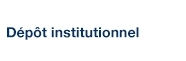# Combinatorial properties of double square tiles

Blondin Massé, Alexandre; Garon, Ariane et Labbé, Sébastien (2013). « Combinatorial properties of double square tiles ». Theoretical Computer Science, 502, pp. 98-117.

Fichier(s) associé(s) à ce document :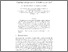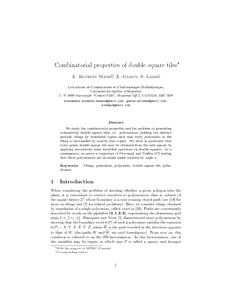Prévisualisation
PDF
Télécharger (608kB)

## Résumé

We study the combinatorial properties and the problem of generating exhaustively double square tiles, i.e. polyominoes yielding two distinct periodic tilings by translated copies such that every polyomino in the tiling is surrounded by exactly four copies. We show in particular that every prime double square tile may be obtained from the unit square by applying successively some invertible operators on double squares. As a consequence, we prove a conjecture of Provençal and Vuillon (2008)  stating that these polyominoes are invariant under rotation by angle π (pi).

Type: Article de revue scientifique Tilings; Generation; Polyomino; Double square tile; Palindromes Faculté des sciences > Département d'informatique Alexandre Blondin Massé 09 mai 2016 14:31 30 mai 2016 14:45 http://archipel.uqam.ca/id/eprint/8432

## Statistiques

Voir les statistiques sur cinq ans...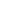RECHERCHER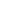PARCOURIR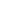LIBRE ACCÈS